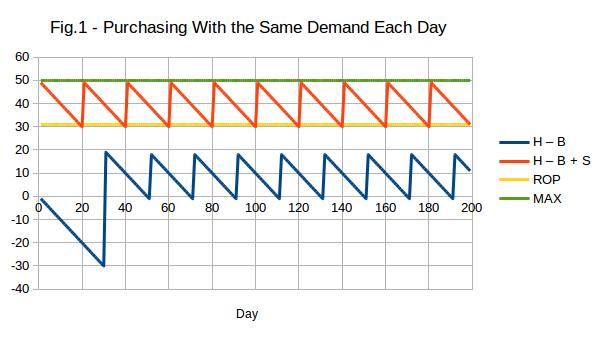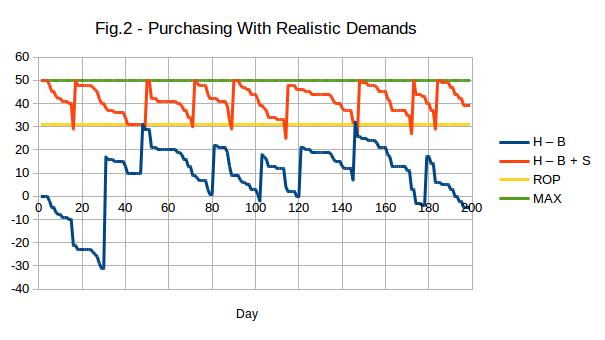Making better use of the data in your inventory system

Consider what I will refer to as the “net quantity” of an item which is the quantity on hand plus the quantity on supplier order minus the quantity on customer back order.

Reordering takes place when the net quantity falls strictly below a level known as the reorder point (ROP). Notice that reordering does not take place when the net quantity is equal to the reorder point. The reason for this is that it is desirable for a reorder point of zero to indicate that the item should not be stocked and should only be ordered when a customers wants it (i.e. when the net quantity is negative).

When an item is reordered, the amount ordered should be such as to increase the net quantity to a level which is referred to as the “maximum” (MAX) or, more descriptively, as the “order-up-to level”.

In Fig.1 below, the reorder point is 31 and the “maximum” level is 50. Items are looked at for possible ordering every day; in other words, the reorder review period is one day. The remainder of the lead time (the time from when the item should be reordered until replenishment) is 30 days. One unit is demanded by customers every day. The letters “H”, “S” and “B” stand for the quantities on hand, on supplier order and on customer back order respectively. Note that H-B+S (orange) is the “net quantity” and that the difference in height between the orange and blue is the quantity on supplier order.H”, “S” and “B” are all treated as being zero initially; hence the customer back orders prior to the arrival of the first shipment.

Note that the reorder point is set to the demand during the lead time which includes the remainder of the reorder review period. Careful examination of the graph will show that there is a shortage of one unit just before each replenishment arrives. This is because the item is not ordered until the next reorder review after the net quantity falls below the reorder point. In this case, the actual lead time can be as much as 31 days consisting of the supplier lead time (30 days) plus the reorder review period (1 day).

Notice that, because of the fact that reordering takes place when the net quantity is 30 (one less than the reorder point), each order quantity is the “maximum” (50) minus the net quantity (30) which gives 20.

There are two aspects of this simulation which are unrealistic. The main one is that demand patterns are rarely as stable as indicated above. In the following graph (Fig.2), everything, including the average daily demand, is the same as in the graph above except that the demand pattern is realistic.Notice the shortages (when the blue curve is below the axis). For this reason, the reorder point needs to be greater than the forecast demand during the reorder review period plus the lead time. The extra amount is known as the “safety stock”. It is the amount of stock which would exist just before replenishment if the demand was perfectly stable.

In practice, there would also be variation in the lead time. This variation increases the amount of safety stock required.

The average order quantity is  MAX – ROP + 1  plus the average demand during half the reorder review period.

Unless an item has frequent shortages, its average stock is approximately the safety stock plus half the average order quantity minus half the average demand during the reorder review period.